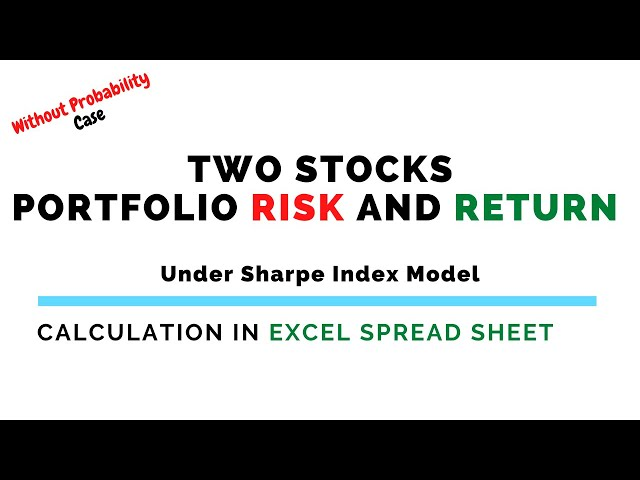﻿case 4 how to calculate portfolio risk return in excel spre

## case 4 how to calculate portfolio risk return in excel spreadsheet

Hi guys! In this video, we'll learn how to calculate two stocks' portfolio risk and return without probability under Sharpe Index Model in Excel spread sheet. I've taken market and two stocks randomly with some of their rate of returns to show the calculation.

Time Stamps:
00:00 Introduction
00:22 Question Discussion
00:54 Solution Sheet
01:32 Step-1&2: Computation Table
02:34 Step-3: Calculation of No. of Periods
02:54 Step-4: Calculation of Individual Risk & Return of Stocks
04:39 Step-5: Calculation of Covariance & Correlation
06:17 Step-6: Calculation of Individual Beta Coefficient of Stocks
06:43 Step-7: Calculation of Portfolio Beta Coefficient of Stocks
07:07 Step-8: Calculation of Portfolio Risk and Return
07:49 Step-9: Conclusion & Decision

Case-1: https://youtu.be/KAU6vnzw_d4
Case-2: https://youtu.be/O_k-Q9NR-S0
Case-3: https://youtu.be/1KHIAbAJMAk

To get the theoretical knowledge,please visit the blog by clicking on the link below:
https://myefinancesheet.blogspot.com/2022/02/measuring-risks.html
https://myefinancesheet.blogspot.com/2022/02/measuring-returns.html

#PortfolioRisk&ReturnAnalysis #SecurityAnalysis #StockSelection